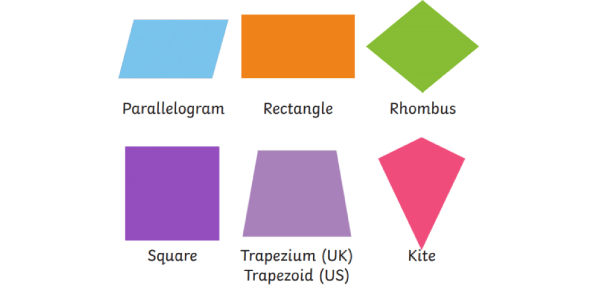10 Questions | Attempts: 9772
ShareSettingsWhen it comes to quadrilaterals, a lot of shapes come to mind. Do you know all the shapes of quadrilaterals well? Take this quadrilateral quiz to see how well you understand and how much you know. It will be a perfect practice test for you to check your memory and what you know about quadrilaterals. Go for it, and try to get a perfect score on this quiz. Also, share the quiz with others who like to learn geometry and its topics.

• 1.
Which is the quadrilateral with exactly one pair of parallel sides?
• A.

Trapezoid

• B.

Squarw

• C.

Rhombus

• D.

Iso trap

• 2.
Which is the parallelogram with 4 right angles?
• A.

Trap

• B.

Rectangle

• C.

Square

• D.

Kite

• E.

Rhombus

• 3.
Name the quadrilateral with 2 distinct pairs of consecutive, congruent sides.
• A.

Parallelogram

• B.

Iso trap

• C.

Trap

• D.

Square

• E.

Kite

• 4.
What is a parallelogram?
• A.

Quadrilateral with at least 1 pair of parallel sides

• B.

• C.

Quadrilateral with 2 pairs of parallel sides

• D.

B and C

• E.

All of the above

• 5.
What is an isosceles trapezoid?
• A.

Trapezod with congruent legs

• B.

Paralleogram with 5 congruent angles

• C.

Trapezoid whose diagonals bisect each other

• D.

Trapezoid with congruent legs

• E.

Square

• 6.
What is a quadrilateral with 4 right angles and an equilateral?
• A.

Rectangle - 120 degrees

• B.

Rectangle

• C.

Square

• D.

Rhombus

• E.

Ambiguous - hydrolysis

• 7.
What parallelogram has diagonals that bisect angles and perpendicular bisectors of each other?
• A.

Square

• B.

Kite

• C.

Rhombus

• D.

Rectangle

• E.

Pentagon

• 8.
Which one of these quadrilaterals has one pair of opposite, parallel sides?
• A.

Square

• B.

Parallelogram

• C.

Trapezoid

• D.

Kite

• E.

Rhombus

• 9.
_________ is any polygon that has 4 sides.
• A.

Rhombus

• B.

Polygon

• C.

Hexagon

• D.

• E.

Trapezoid

• 10.
Which word best describes a figure that contains 4 right angles and 4 equal sides?
• A.

Rhombus

• B.

Square

• C.

Rectangle

• D.

Isosceles Triangle

## Related TopicsBack to top
×

Wait!
Here's an interesting quiz for you.